Methodology development

(diff) ← Older revision | Latest revision (diff) | Newer revision → (diff)

Computational Developments

The chief mission of our group is to apply state-of-the-art computational methods top problems in materials science at atomic scale. Mainly, this means accurate description of electronic structures and states in solids, nanostructures, and point defects therein. In certain problems where existing computational tools were inadequate for our goals, we have initiated the development or implementation of new ones. This means standalone codes and modules for larger packages to calculate the electronic structure of strongly correlated materials, excitation and impact ionization rates in them, Auger recombination rates for defects in solids, and spin-dependent parameters such as hyperfine tensor or zero-field splitting tensor associated with electron spin-electron spin interaction. Modules have been developed for ab-initio plane-wave packages such as Quantum Espresso and VASP, with the help of core developers (Dario Rocca at the Giulia Galli group for Quantum Espresso and Martijn Marsman at the Georg Kresse group for VASP).

Impact ionization and Auger recombination rates

Impact ionization rates may be well-estimated by the imaginary part of the self-energy, which can be calculated within the so-called GW approximation <bib id="Kotani"/>. With little modification of the official VASP implementation of GW code, we applied this method for a strongly correlated material <bib id="PhysRevB.90.165142"/>. Another treatment of this problem uses integrals of type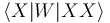$\langle X | W | XX \rangle$

where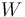$W$ is the screened Coulomb interaction appearing in the GW formalism, and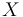$X$ and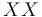$XX$ are exciton and biexciton wavefunctions. We have developed a standalone code (in real space) to calculate these rates. We have also integrated our tool into the Quantum Espresso code, in the frequency space with full treatment of$W$ <bib id="PhysRevB.87.155402"/>. We have applied this theory to calculate the impact ionization rates in silicon nanoparticles <bib id="PhysRevLett.110.046804"/>, or the Auger recombination rates in the nitrogen-vacancy center (NV) in diamond <bib id="PhysRevLett.110.167402"/>.

Hyperfine tensor

Hyperfine interaction occurs between the spin of the electrons of a molecular system and the nuclear spins. For defects, the spin of electrons means the net total spin of electrons localized around the defect. For example NV has a total spin of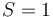$S = 1$.

We have implemented the spin-density contribution of atomic core electrons (closed-shell electrons) via the Fermi contact term into the hyperfine tensor calculation in collaboration with Martijn Marsman from the University of Vienna. We have shown that this effect can be enormously large for some point defects, in contrast to previous expectations. Moreover, we have found that the usage of HSE06 hybrid density functional in electronic system calculations, together with the contribution of core spin polarization provides accurate results on prominent point defects in various semiconductors <bib id="PhysRevB.88.075202"/>.

Zero-field splitting tensor

The electron-spin sublevels of a high-spin structure, e.g. a defect (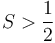$S>\frac12$) may be nondegenerate even in the absence of external magnetic field. This phenomenon is called zero-field splitting. It is caused by the electron spin-electron spin interaction, as the anisotropy of dipole-dipole interaction makes the shape of electron cloud relevant with respect to the direction of total spin. In total, the energy of zero-field splitting is quadratic in the spin,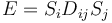$E = S_iD_{ij}S_j$, where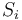$S_i$ are spin components, and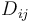$D_{ij}$ are components of the zero-field splitting tensor. We have developed the elements of calculating this zero-field splitting tensor within the projector-augmentation-wave (PAW) framework <bib id="bodrog2014spin"/>. It has been recently implemented into the VASP code, and is in testing phase.

Hybrid density functional with orbital-dependent screening

Hybrid functionals serve as a powerful practical tool in different fields of computational physics and quantum chemistry. On the other hand, non-universality of practical exchange-correlation functionals, particularly their weak applicability to the case of correlated d and f orbitals is still a question, and development in this field still needs considerable effort. We have formulated the on-site occupation-dependent exchange correlation energy and effective potential of hybrid functionals for localized states, and we have connected them to the on-site correction term of the DFT + U method. The resulting formula indicates that the screening of onsite electron repulsion is governed by the ratio of exact exchange in the hybrid functional. Our derivation also provides a theoretical justification for adding a DFT + U-like on-site potential to hybrid DFT calculations in order to resolve issues caused by overscreening of localized states <bib id="PhysRevB.87.205201"/> <bib id="PhysRevB.90.035146"/>.

<biblist />